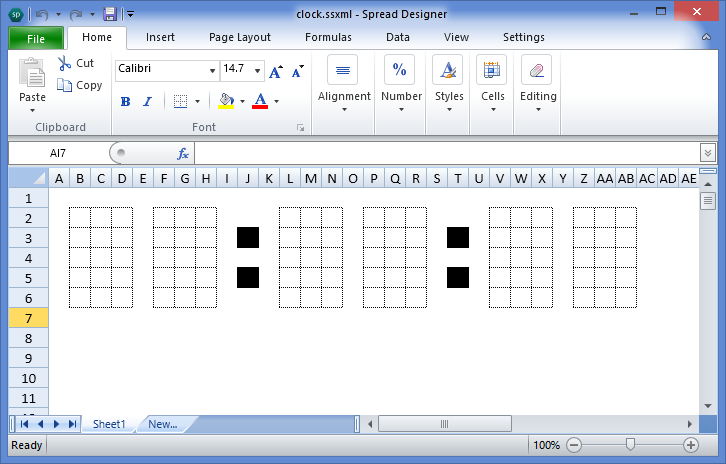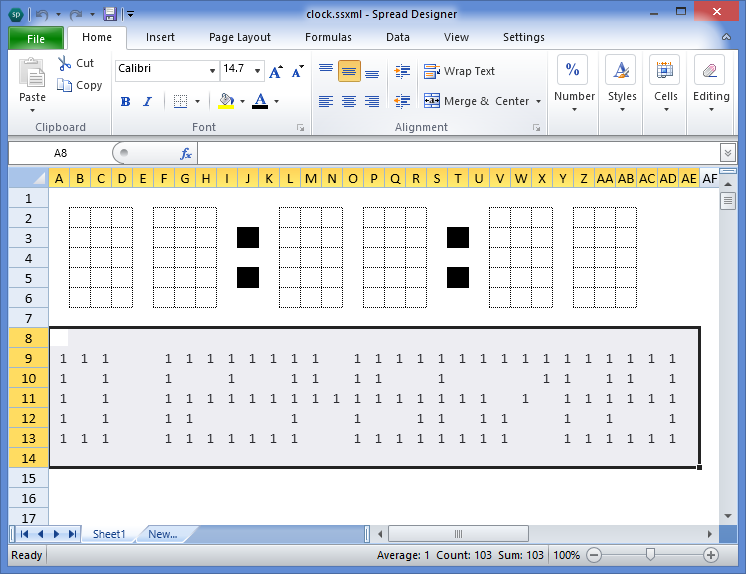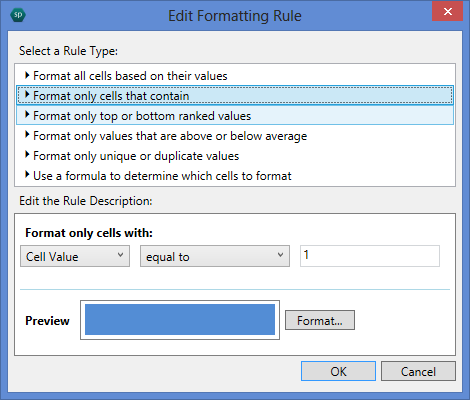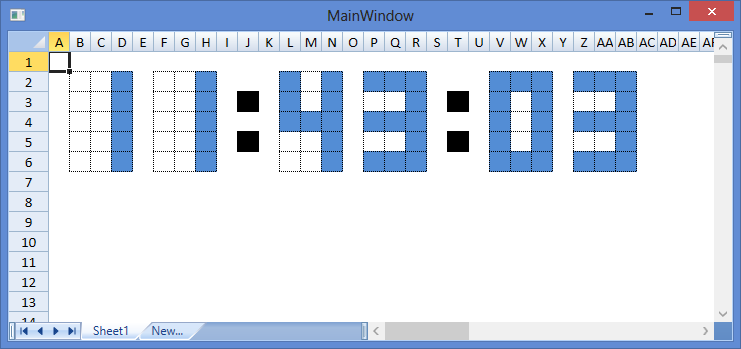=LEFT(TEXT(HOUR(NOW()),"00"))

=OFFSET(DigitPattern,ROW()-ROW(B2),COLUMN()-COLUMN(B2)+A1*3)```public MainPage()
{
this.InitializeComponent();
DispatcherTimer dt = new DispatcherTimer();
dt.Interval = TimeSpan.FromSeconds(1);
dt.Tick += (sender, e) => { if (this.gcSpreadSheet1.ActiveSheet != null) this.gcSpreadSheet1.ActiveSheet.Recalculate(); };
dt.Start();
} ```WpfApplication1.zip (21.51 kb)